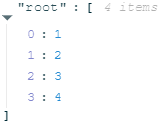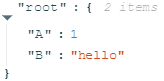# Visualizing Data

This markdown was exported from Data-Forge Notebook

# Examples of visualization

There's many ways to visualize your data in Data-Forge Notebook, lets look at a few here.

## Plain ole' console logging

Use `console.log` to output whatever you want:

```const data = "Whatever you want to log!";
console.log(data);```
``````Whatever you want to log!

``````

## JavaScript array

We can use the `display` function for formatted data visualization.

We can use it to visualize JavaScript arrays:

```const data = [1, 2, 3, 4];
display(data);```## JavaScript object

We can also use the `display` function to visualize JavaScript objects:

```const data = { A: 1, B: "hello" };
display(data);```## JavaScript object as table

We can use `display.table` to visualize a JavaScript object as tabular data:

```const data = { A: 1, B: "hello" };
display.table(data);```
Property Value
A 1
B hello

We can also use display.table to visualize a JavaScript array as tabular data.

```const data = [{ A: 1, B: "Hello" }, { A: 2, B: "Computer!" }];
display.table(data);```
index A B
0 1 Hello
1 2 Computer!

## HTML!

Then there's `display.html` that we can use to render formatted HTML:

```const data = "<h1>This is HTML</h1>";
display.html(data);```

# This is HTML

## Tabular data

The `display` function automatically formats a dataframe as tabular data!

```const dataForge = require('data-forge');
require('data-forge-fs');
index Name Sex Age Height (in) Weight (lbs)
0 Alex M 41 74 170
1 Bert M 42 68 166
2 Carl M 32 70 155
3 Dave M 39 72 167
4 Elly F 30 66 124

## Charts: Plot any ole JavaScript data!

Easily plot data from JavaScript or JSON using `display.plot`:

```const data = [50, 20, 10, 40, 15, 25];
display.plot(data);```
```const data = [{ D1: 50, D2: 30 }, { D1: 20, D2: 200 }, { D1: 10, D2: 100 }, { D1: 40, D2: 400 }];
display.plot(data);```

## Charts!

The `display` function knows how render a plot created from a dataframe!

```const dataForge = require('data-forge');
require('data-forge-fs');
require('data-forge-plot');
display(data.plot());```## More charts!

Want to see more charts?

## More coming soon!

More visualization are coming in future editions of Data-Forge Notebook, for example:

• Geographic data
• Images
• Node.js buffers
• Vectors, matrices and maths formulas
• Colors

Email and let me know what else could be added!

This markdown was exported from Data-Forge Notebook

##### Clone this wiki locally
You can’t perform that action at this time.